# Lesson Overview 11 2 Applying Mendels Principles Applying

• Slides: 13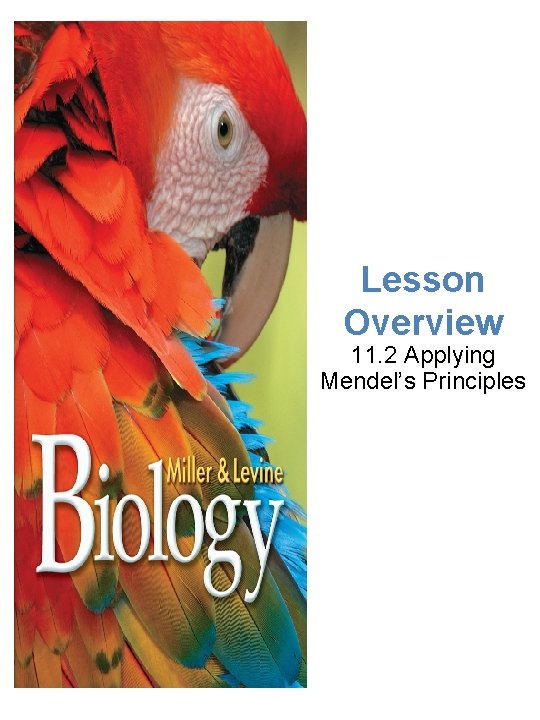Lesson Overview 11. 2 Applying Mendel’s Principles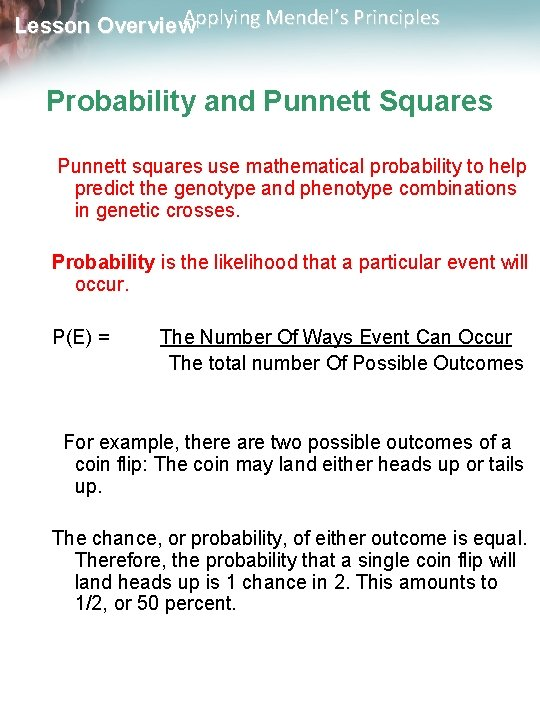Applying Mendel’s Principles Lesson Overview Probability and Punnett Squares Punnett squares use mathematical probability to help predict the genotype and phenotype combinations in genetic crosses. Probability is the likelihood that a particular event will occur. P(E) = The Number Of Ways Event Can Occur The total number Of Possible Outcomes For example, there are two possible outcomes of a coin flip: The coin may land either heads up or tails up. The chance, or probability, of either outcome is equal. Therefore, the probability that a single coin flip will land heads up is 1 chance in 2. This amounts to 1/2, or 50 percent.Applying Mendel’s Principles Lesson Overview Probability and Punnett Squares If you flip a coin three times in a row, what is the probability that it will land heads up every time? Each coin flip is an independent event, with a one chance in two probability of landing heads up. Therefore, the probability of flipping three heads in a row is: 1/2 × 1/2 = 1/8Applying Mendel’s Principles Lesson Overview Probability and Punnett Squares As you can see, you have 1 chance in 8 of flipping heads three times in a row. Past outcomes do not affect future ones. Just because you’ve flipped 3 heads in a row does not mean that you’re more likely to have a coin land tails up on the next flip.Applying Mendel’s Principles Lesson Overview Using Segregation to Predict Outcomes The way in which alleles segregate during gamete formation is every bit as random as a coin flip. Therefore, the principles of probability can be used to predict the outcomes of genetic crosses.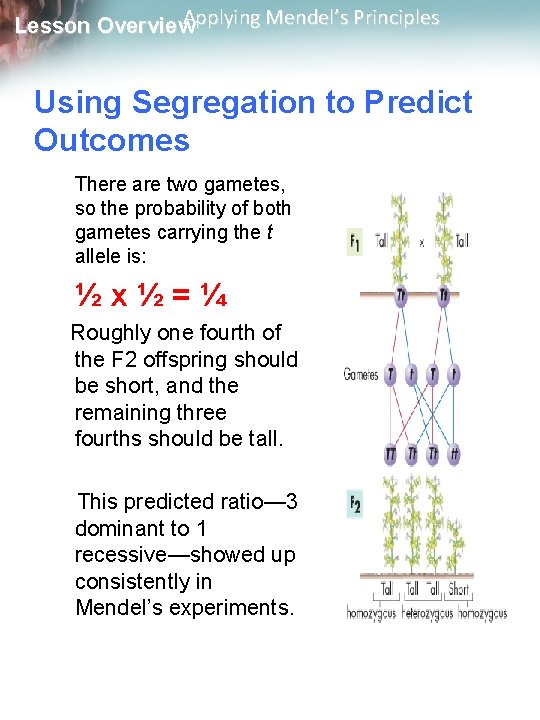Applying Mendel’s Principles Lesson Overview Using Segregation to Predict Outcomes There are two gametes, so the probability of both gametes carrying the t allele is: ½x½=¼ Roughly one fourth of the F 2 offspring should be short, and the remaining three fourths should be tall. This predicted ratio— 3 dominant to 1 recessive—showed up consistently in Mendel’s experiments.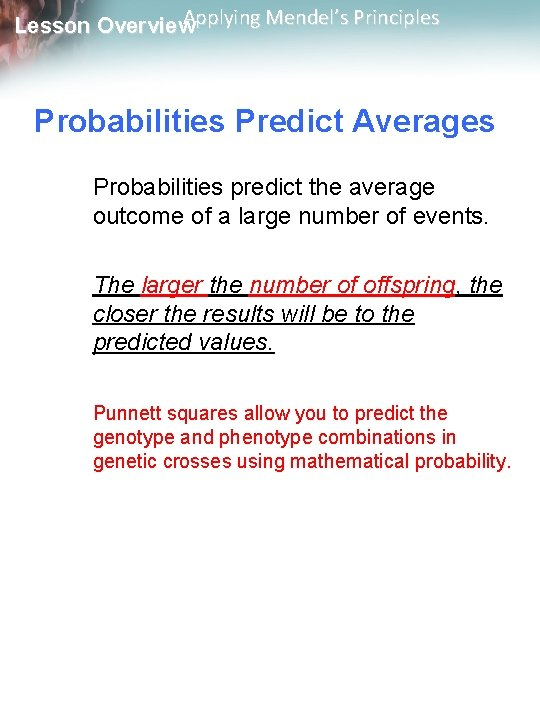Applying Mendel’s Principles Lesson Overview Probabilities Predict Averages Probabilities predict the average outcome of a large number of events. The larger the number of offspring, the closer the results will be to the predicted values. Punnett squares allow you to predict the genotype and phenotype combinations in genetic crosses using mathematical probability.Applying Mendel’s Principles Lesson Overview How To Make a Punnett Square Draw a table with enough spaces for each pair of gametes from each parent. Enter the genotypes of the gametes produced by both parents on the top and left sides of the table.Applying Mendel’s Principles Lesson Overview How To Make a Punnett Square Fill in the table by combining the gametes’ genotypes.Applying Mendel’s Principles Lesson Overview How To Make a Punnett Square Determine the genotypes and phenotypes of each offspring. Calculate the percentage or ratios of each. In this example, three fourths of the chicks will have large beaks, but only one in two will be heterozygous.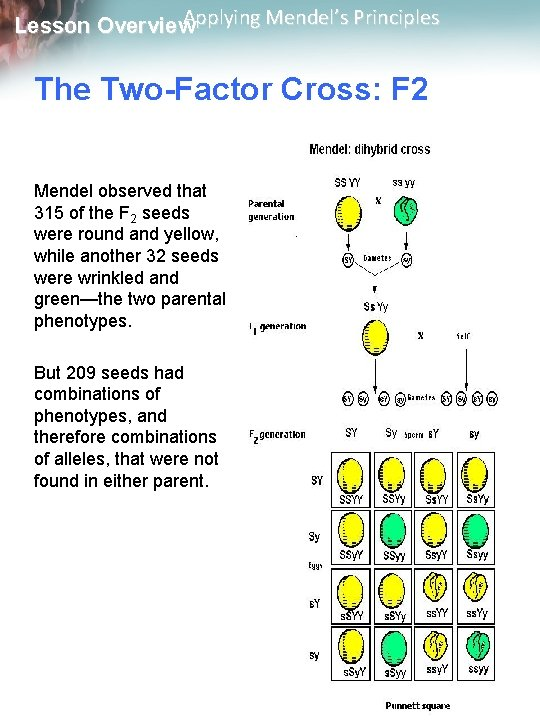Applying Mendel’s Principles Lesson Overview The Two-Factor Cross: F 2 Mendel observed that 315 of the F 2 seeds were round and yellow, while another 32 seeds were wrinkled and green—the two parental phenotypes. But 209 seeds had combinations of phenotypes, and therefore combinations of alleles, that were not found in either parent.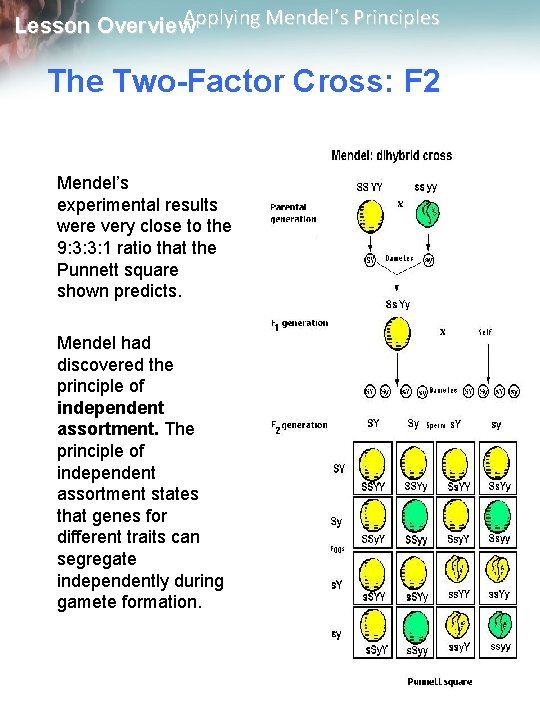Applying Mendel’s Principles Lesson Overview The Two-Factor Cross: F 2 Mendel’s experimental results were very close to the 9: 3: 3: 1 ratio that the Punnett square shown predicts. Mendel had discovered the principle of independent assortment. The principle of independent assortment states that genes for different traits can segregate independently during gamete formation.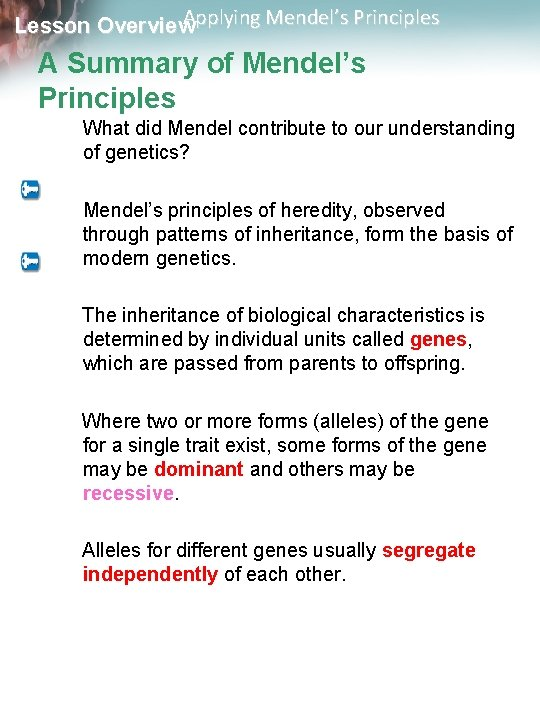Applying Mendel’s Principles Lesson Overview A Summary of Mendel’s Principles What did Mendel contribute to our understanding of genetics? Mendel’s principles of heredity, observed through patterns of inheritance, form the basis of modern genetics. The inheritance of biological characteristics is determined by individual units called genes, which are passed from parents to offspring. Where two or more forms (alleles) of the gene for a single trait exist, some forms of the gene may be dominant and others may be recessive. Alleles for different genes usually segregate independently of each other.